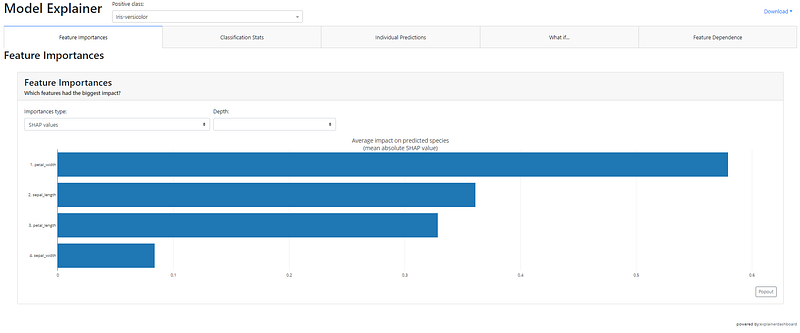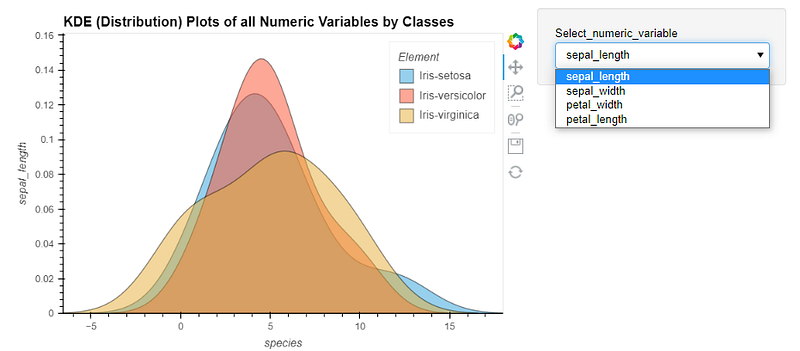Comment on page

# PyCaret 2.3.6 is Here! Learn What’s New?

PyCaret 2.3.6 is Here! Learn What’s New? From EDA to Deployment to AI Fairness — By far the biggest release of PyCaret

### 🚀 Introduction

PyCaret is an open-source, low-code machine learning library in Python that automates machine learning workflows. It is an end-to-end machine learning and model management tool that speeds up the experiment cycle exponentially and makes you more productive.
By far PyCaret 2.3.6 is the biggest release in terms of the new features and functionalities. This article demonstrates the use of new functionalities added in the recent release of PyCaret 2.3.6.

### 💻 Installation

Installation is easy and will only take a few minutes. PyCaret’s default installation from pip only installs hard dependencies as listed in the requirements.txt file.
pip install pycaret
To install the full version:
pip install pycaret[full]

### 📈 Dashboard

This function will generate the interactive dashboard for a trained model. The dashboard is implemented using the ExplainerDashboard.
from pycaret.datasets import get_data
data = get_data('iris')
# init setupfrom pycaret.classification import *
s = setup(data, target = 'species', session_id = 123)
# train model
lr = create_model('lr')
# generate dashboard
dashboard(lr)Video Demo:

### 📊 Exploratory Data Analysis (EDA)

This function will generate automated EDA using the AutoViz integration.
from pycaret.datasets import get_data
data = get_data('iris')
# init setup
from pycaret.classification import *
s = setup(data, target = 'species', session_id = 123)
# generate EDA
eda()Video Demo:

### 🚊 Convert Model

This function will transpile trained machine learning models into native inference scripts in different programming languages (Python, C, Java, Go, JavaScript, Visual Basic, C#, PowerShell, R, PHP, Dart, Haskell, Ruby, F#). This functionality is very useful if you want to deploy models into environments where you can’t install your normal Python stack to support model inference.
from pycaret.datasets import get_data
data = get_data('iris')
# init setup
from pycaret.classification import *
s = setup(data, target = 'species', session_id = 123)
# train model
lr = create_model('lr')
# convert model
lr_java = convert_model(lr, language = 'java')
print(lr_java)Video Demo:

### ☑️ Check Fairness

There are many approaches to conceptualizing fairness. This new function follows the approach known as group fairness, which asks: Which groups of individuals are at risk for experiencing harm. This function provides fairness-related metrics between different groups (also called subpopulations).
from pycaret.datasets import get_data
data = get_data('income')
# init setup
from pycaret.classification import *
s = setup(data, target = 'income >50K', session_id = 123)
# train model
lr = create_model('lr')
# check fairness
check_fairness(lr, sensitive_features = ['race'])Video Demo:

### 📩 Create Web API

This function will create a POST API for the ML pipeline for inference using FastAPI framework. It only creates the API and doesn’t run it automatically.
from pycaret.datasets import get_data
data = get_data('iris')
# init setup
from pycaret.classification import *
s = setup(data, target = 'species', session_id = 123)
# train model
lr = create_model('lr')
# create API
create_api(lr, 'my_first_api')
# Run the API
!python my_first_api.py### 🚢 Create Docker

This function will create a `Dockerfile`and `requirements`file for your API end-point.
from pycaret.datasets import get_data
data = get_data('iris')
# init setup
from pycaret.classification import *
s = setup(data, target = 'species', session_id = 123)
# train model
lr = create_model('lr')
# create API
create_api(lr, 'my_first_api')
# create Docker
create_docker('my_first_api')Video Demo:

### 💻 Create Web Application

This function creates a basic Gradio web app for inference. It will later be expanded for other app types such as Streamlit.
from pycaret.datasets import get_data
data = get_data('iris')
# init setup
from pycaret.classification import *
s = setup(data, target = 'species', session_id = 123)
# train model
lr = create_model('lr')Video Demo:

### 🎰 Monitor Drift of ML Models

A new parameter called `drift_report` is added to the `predict_model` function that generates the drift report using Evidently AI framework. At the moment this functionality is in experimental mode and will only work on test data. Later on, it will be expanded for production use.
from pycaret.datasets import get_data
data = get_data('iris')
# init setup
from pycaret.classification import *
s = setup(data, target = 'species', session_id = 123)
# train model
lr = create_model('lr')
# generate report
preds = predict_model(lr, drift_report = True)Video Demo:

### 🔨 Plot Model is now more configurable

`plot_model` function is PyCaret is now more configurable. For example, previously if you wanted to see percentages in Confusion Matrix instead of absolute numbers, it wasn’t possible, or if you want to change the color map of visuals, it wasn’t possible. Now it is possible with the new parameter `plot_kwargs` in the `plot_model` function. See example:
from pycaret.datasets import get_data
data = get_data('iris')
# init setup
from pycaret.classification import *
s = setup(data, target = 'species', session_id = 123)
# train model
lr = create_model('lr')
# plot model (without plot kwargs)
plot_model(lr, plot = 'confusion_matrix')
# plot model (with plot kwargs)
plot_model(lr, plot = 'confusion_matrix', plot_kwargs = {'percent' : True})### 🏆Optimize Threshold

This is not a new function but it was completely revamped in 2.3.6. This function is to optimize the probability threshold for binary classification problems. Previously you had to pass cost function as `true_positive` , `false_positive` , `true_negative` , `false_negative` in this function and now it automatically picks up all the metrics including the custom ones from your active experiment run.
from pycaret.datasets import get_data
data = get_data('blood')
# init setup
from pycaret.classification import *
s = setup(data, target = 'Class', session_id = 123)
# train model
lr = create_model('lr')
# optimize threshold
optimize_threshold(lr)### 📚 New Documentation

The biggest and hardest of all is the completely new documentation. This is a single source of truth for everything related to PyCaret, from official tutorials to release notes and from API ref to community contributions. Take a video tour:
Finally, if you want to take the tour of all new functionalities added in 2.3.6, watch this 10 minutes video:
To learn about all the other changes, bug fixes, and minor updates in PyCaret 2.3.6, check out the detailed release notes.